Documentation

SimBiology.Scenarios

Simulation scenarios

Description

SimBiology.Scenarios is an object that lets you generate different simulation scenarios based on different sample values of model quantities. You can combine these quantities with different doses or variants and simulate various scenarios to explore model behaviors under different experimental conditions and dosing regimens.

Creation

Description

example

sObj = SimBiology.Scenarios returns a Scenarios object sObj that contains no entries.

example

sObj = SimBiology.Scenarios(name,content) returns a Scenarios object sObj with one entry. name is the name of a model quantity or the name of a group of variants or doses for scenario generation. content contains the corresponding numeric values for the model quantity or a vector of variant objects or vector of dose objects.

example

sObj = SimBiology.Scenarios(quantityNames,probDist,Name,Value) specifies to generate the sample values for one or more model quantities quantityNames from the joint probability distribution probDist. Specify additional options for the probability distributions and sampling method using one or more name-value pair arguments. To specify the probability distributions, you must have Statistics and Machine Learning Toolbox™.

Input Arguments

expand all

Entry name, specified as a character vector or string.

You can set the entry name to the name of a model quantity (species, parameter, or compartment). Alternatively, you can define a name for a group of doses or variants to be included in the sample (scenarios) generation.

Example: "k1"

Data Types: char | string

Model quantity values, or a vector of doses or variants, specified as a numeric vector, vector of RepeatDose or ScheduleDose objects, or vector of variant objects.

If you specify a quantity name for the name input argument, set content to a numeric vector.

If you specify a name for a group of doses or variants, set content to a vector of dose objects or vector of variant objects.

Example: [0.5,1,1.5]

Names of model quantities for the sample (scenario) generation, specified as a character vector, string, string vector, or cell array of character vectors.

Example: ["k12","k21"]

Data Types: char | string | cell

Probability distributions to generate sample values for model quantities, specified as a vector of probability distribution objects, character vector, string, string vector, or cell array of character vectors containing the names of supported probability distributions. To specify the probability distributions, you must have Statistics and Machine Learning Toolbox.

Use the makedist function to create distribution objects. For a list of supported distributions, see distname (Statistics and Machine Learning Toolbox).

Example: [pd1,pd2]

Name-Value Pair Arguments

Specify optional comma-separated pairs of Name,Value arguments. Name is the argument name and Value is the corresponding value. Name must appear inside quotes. You can specify several name and value pair arguments in any order as Name1,Value1,...,NameN,ValueN.

Example: 'Number',10 specifies to generate 10 samples.

Number of samples to draw from probability distributions, specified as the comma-separated pair consisting of 'Number' and a positive scalar. The default value [] means that the function infers the number of samples from other entries. If the number cannot be inferred, the number is set to 2.

Example: 'Number',5

Rank correlation matrix for the joint probability distribution, specified as the comma-separated pair consisting of 'RankCorrelation' and a numeric matrix. The default behavior is that when both 'RankCorrelation' and 'Covariance' are set to [], SimBiology.Scenarios draws uncorrelated samples from the joint probability distribution.

You cannot specify 'RankCorrelation' if 'Covariance' is set. The number of columns in the matrix must match the number of specified distributions. The matrix must be symmetric with diagonal values of 1. All of its eigenvalues must also be positive.

Example: 'RankCorrelation',[1 0.3;0.3 1]

Mean values of quantities, specified as the comma-separated pair consisting of 'Mean' and a numeric vector.

You can specify mean values for normal distributions only. The number of mean values must equal the number of specified probability distributions.

Example: 'Mean',[0.5,1.5]

Covariance matrix for the joint probability distribution, specified as the comma-separated pair consisting of 'Covariance' and a numeric matrix. The default behavior is that if both 'RankCorrelation' and 'Covariance' are set to [], SimBiology.Scenarios draws uncorrelated samples from the joint probability distribution. You cannot specify 'Covariance' if you specify 'RankCorrelation'.

You can specify the covariance matrix for normal distributions only. The number of columns in the matrix must match the number of specified distributions. All of its eigenvalues must also be nonnegative.

Example: 'Covariance',[0.25 0.15;0.15 0.25]

Sampling method, specified as the comma-separated pair consisting of 'SamplingMethod' and a character vector or string. Depending on whether probability distributions with 'RankCorrelation' or normal distributions with 'Covariance' are specified, the sampling techniques differ.

If an entry contains a (joint) normal distribution with Covariance specified, the sampling methods are:

• 'random' – Draw random samples from the specified normal distribution using mvnrnd.

• 'lhs' – Draw Latin hypercube samples from the specified normal distributions using lhsnorm. For details, see Generating Quasi-Random Numbers (Statistics and Machine Learning Toolbox).

If an entry contains a (joint) distribution with no Covariance specified, the sampling methods are:

• 'random' – Draw random samples from the specified probability distributions using random.

• 'lhs' – Draw Latin hypercube samples from the specified probability distributions using an algorithm similar to lhsdesign. This approach is a more systematic space-filling approach than random sampling. For details, see Generating Quasi-Random Numbers (Statistics and Machine Learning Toolbox).

• 'copula' – Draw random samples using a copula (Statistics and Machine Learning Toolbox). Use this option to impose correlations between samples using copulas.

• 'sobol' – Use the sobol sequence (sobolset) which is transformed using the inverse cumulative distribution function (icdf) of the specified probability distributions. Use this method for highly systematic space-filling. For details, see Generating Quasi-Random Numbers (Statistics and Machine Learning Toolbox).

• 'halton' – Use the halton sequence (haltonset) which is transformed using the inverse cumulative distribution function (icdf) of the specified probability distributions. For details, see Generating Quasi-Random Numbers (Statistics and Machine Learning Toolbox).

If no Covariance is specified, SimBiology.Scenarios essentially performs two steps. The first step is to generate samples using one of the above sampling methods. For lhs, sobol, and halton methods, the generated uniform samples are transformed to samples from the specified distribution using the inverse cumulative distribution function icdf. Then, as the second step, the samples are correlated using the Iman-Conover algorithm if RankCorrelation is specified. For random, the samples are drawn directly from the specified distributions and the samples are then correlated using the Iman-Conover algorithm.

Example: 'SamplingMethod','lhs'

Properties

expand all

This property is read-only.

Combination expression summarizing the combination of entries in the object, specified as a character vector. The plus + sign indicates the elementwise combination, and the cross x sign indicates the cartesian combination. For details, see Combine Simulation Scenarios in SimBiology.

Example: '(k1 + k2 + k3) x doses'

Data Types: char

Number of entries in the scenarios object, specified as a positive integer.

Example: 4

Data Types: double

Seed for random number generation to obtain reproducible scenarios, specified as a nonnegative integer smaller than 232. The default value [] means that the generated scenarios will be different every time the generate function is called unless you set the random seed before calling the function or use reproducible sequences such as Sobol or Halton.

Example: 10

Data Types: double

Object Functions

 add Add quantity values, doses, or variants to SimBiology.Scenarios object getEntry Get entry contents from SimBiology.Scenarios object updateEntry Update entry contents from SimBiology.Scenarios object rename Rename entry from SimBiology.Scenarios object remove Remove entries from SimBiology.Scenarios object verify Verify SimBiology.Scenarios object generate Generate scenarios from SimBiology.Scenarios object and return table getNumberScenarios Return number of scenarios from SimBiology.Scenarios object

Examples

collapse all

Load the model of glucose-insulin response. For details about the model, see the Background section in Simulating the Glucose-Insulin Response.

The model contains different parameter values and initial conditions that represents different insulin impairments (such as Type 2 diabetes, low insulin sensitivity, and so on) stored in five variants.

variants = getvariant(m1)
variants =
SimBiology Variant Array

Index:  Name:             Active:
1       Type 2 diabetic   false
2       Low insulin se... false
3       High beta cell... false
4       Low beta cell ... false
5       High insulin s... false

Suppress an informational warning that is issued during simulations.

warnSettings = warning('off','SimBiology:DimAnalysisNotDone_MatlabFcn_Dimensionless');

Select a dose that represents a single meal of 78 grams of glucose.

singleMeal = sbioselect(m1,'Name','Single Meal');

Create a Scenarios object to represent different initial conditions combined with the dose. That is, create a scenario object where each variant is paired (or combined) with the dose, for a total of five simulation scenarios.

sObj = SimBiology.Scenarios;
ans =
Scenarios (5 scenarios)

Name            Content          Number
________    ___________________    ______

Entry 1      variants    SimBiology variants      5
x Entry 2    dose        SimBiology dose          1

sObj contains two entries. Use the generate function to combine the entries and generate five scenarios. The function returns a scenarios table, where each row represents a scenario and each column represents an entry of the Scenarios object.

scenariosTbl = generate(sObj)
scenariosTbl=5×2 table
variants                       dose
________________________    ___________________________

[1×1 SimBiology.Variant]    [1×1 SimBiology.RepeatDose]
[1×1 SimBiology.Variant]    [1×1 SimBiology.RepeatDose]
[1×1 SimBiology.Variant]    [1×1 SimBiology.RepeatDose]
[1×1 SimBiology.Variant]    [1×1 SimBiology.RepeatDose]
[1×1 SimBiology.Variant]    [1×1 SimBiology.RepeatDose]

Change the entry name of the first entry.

rename(sObj,1,'Insulin Impairements')
ans =
Scenarios (5 scenarios)

Name                  Content          Number
____________________    ___________________    ______

Entry 1      Insulin Impairements    SimBiology variants      5
x Entry 2    dose                    SimBiology dose          1

Create a SimFunction object to simulate the generated scenarios. Use the Scenarios object as the input and specify the plasma glucose and insulin concentrations as reponses (outputs of the function to be plotted). Specify [] for the dose input argument since the Scenarios object already has the dosing information.

f = createSimFunction(m1,sObj,{'[Plasma Glu Conc]','[Plasma Ins Conc]'},[])
f =
SimFunction

Parameters:

Name                Value         Type                            Units
____________________________    ______    _____________    ___________________________________________

{'Plasma Volume (Glu)'     }      1.88    {'parameter'}    {'deciliter'                              }
{'k1'                      }     0.065    {'parameter'}    {'1/minute'                               }
{'k2'                      }     0.079    {'parameter'}    {'1/minute'                               }
{'Plasma Volume (Ins)'     }      0.05    {'parameter'}    {'liter'                                  }
{'m1'                      }      0.19    {'parameter'}    {'1/minute'                               }
{'m2'                      }     0.484    {'parameter'}    {'1/minute'                               }
{'m4'                      }    0.1936    {'parameter'}    {'1/minute'                               }
{'m5'                      }    0.0304    {'parameter'}    {'minute/picomole'                        }
{'m6'                      }    0.6469    {'parameter'}    {'dimensionless'                          }
{'Hepatic Extraction'      }       0.6    {'parameter'}    {'dimensionless'                          }
{'kmax'                    }    0.0558    {'parameter'}    {'1/minute'                               }
{'kmin'                    }     0.008    {'parameter'}    {'1/minute'                               }
{'kabs'                    }    0.0568    {'parameter'}    {'1/minute'                               }
{'kgri'                    }         0    {'parameter'}    {'1/minute'                               }
{'f'                       }       0.9    {'parameter'}    {'dimensionless'                          }
{'a'                       }         0    {'parameter'}    {'1/milligram'                            }
{'b'                       }      0.82    {'parameter'}    {'dimensionless'                          }
{'c'                       }         0    {'parameter'}    {'1/milligram'                            }
{'d'                       }      0.01    {'parameter'}    {'dimensionless'                          }
{'Stomach Glu After Dosing'}        78    {'parameter'}    {'gram'                                   }
{'kp1'                     }       2.7    {'parameter'}    {'milligram/minute'                       }
{'kp2'                     }    0.0021    {'parameter'}    {'1/minute'                               }
{'kp3'                     }     0.009    {'parameter'}    {'(milligram/minute)/(picomole/liter)'    }
{'kp4'                     }    0.0618    {'parameter'}    {'(milligram/minute)/picomole'            }
{'ki'                      }    0.0079    {'parameter'}    {'1/minute'                               }
{'[Ins Ind Glu Util]'      }         1    {'parameter'}    {'milligram/minute'                       }
{'Vm0'                     }    2.5129    {'parameter'}    {'milligram/minute'                       }
{'Vmx'                     }     0.047    {'parameter'}    {'(milligram/minute)/(picomole/liter)'    }
{'Km'                      }    225.59    {'parameter'}    {'milligram'                              }
{'p2U'                     }    0.0331    {'parameter'}    {'1/minute'                               }
{'K'                       }      2.28    {'parameter'}    {'picomole/(milligram/deciliter)'         }
{'alpha'                   }      0.05    {'parameter'}    {'1/minute'                               }
{'beta'                    }      0.11    {'parameter'}    {'(picomole/minute)/(milligram/deciliter)'}
{'gamma'                   }       0.5    {'parameter'}    {'1/minute'                               }
{'ke1'                     }    0.0005    {'parameter'}    {'1/minute'                               }
{'ke2'                     }       339    {'parameter'}    {'milligram'                              }
{'Basal Plasma Glu Conc'   }     91.76    {'parameter'}    {'milligram/deciliter'                    }
{'Basal Plasma Ins Conc'   }     25.49    {'parameter'}    {'picomole/liter'                         }

Observables:

Name                Type                 Units
_____________________    ___________    _______________________

{'[Plasma Glu Conc]'}    {'species'}    {'milligram/deciliter'}
{'[Plasma Ins Conc]'}    {'species'}    {'picomole/liter'     }

Dosed:

TargetName       TargetDimension
__________    _____________________

{'Dose'}     {'Mass (e.g., gram)'}

Simulate the model for 24 hours and plot the simulation data. The data contains five runs, where each run represents a scenario in the Scenarios object.

sd = f(sObj,24);
sbioplot(sd)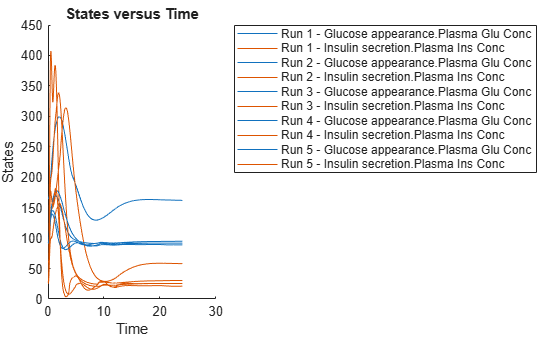ans =
Axes (SbioPlot) with properties:

XLim: [0 25]
YLim: [0 450]
XScale: 'linear'
YScale: 'linear'
GridLineStyle: '-'
Position: [73.8000 47.2000 342.2000 342.8000]
Units: 'pixels'

Show all properties

If you have Statistics and Machine Learning Toolbox™, you can also draw sample values for model quantities from various probability distributions. For instance, suppose that the parameters Vmx and kp3, which are known for the low and high insulin sensitivity, follow the lognormal distribution. You can generate sample values for these parameters from such a distribution, and perform a scan to explore model behavior.

Define the lognormal probability distribution object for Vmx.

pd_Vmx = makedist('lognormal')
pd_Vmx =
LognormalDistribution

Lognormal distribution
mu = 0
sigma = 1

By definition, the parameter mu is the mean of logarithmic values. To vary the parameter value around the base (model) value of the parameter, set mu to log(model_value). Set the standard deviation to 0.2.

Vmx = sbioselect(m1,'Name','Vmx');
pd_Vmx.mu = log(Vmx.Value);
pd_Vmx.sigma = 0.2
pd_Vmx =
LognormalDistribution

Lognormal distribution
mu = -3.05761
sigma =      0.2

Similarly define the probability distribution for kp3.

pd_kp3 = makedist('lognormal');
kp3 = sbioselect(m1,'Name','kp3');
pd_kp3.mu = log(kp3.Value);
pd_kp3.sigma = 0.2
pd_kp3 =
LognormalDistribution

Lognormal distribution
mu = -4.71053
sigma =      0.2

Now define a joint probability distribution to draw sample values for Vmx and kp3, with a rank correlation to specify some correlation between these two parameters. Note that this correlation assumption is for the illustration purposes of this example only and may not be biologically relevant.

First remove the variants entry (entry 1) from sObj.

remove(sObj,1)
ans =
Scenarios (1 scenarios)

Name        Content        Number
____    _______________    ______

Entry 1    dose    SimBiology dose      1

Add an entry that defines the joint probability distribution with a rank correlation matrix.

ans =
Scenarios (2 scenarios)

Name           Content              Number
____    ______________________    ___________

Entry 1         dose    SimBiology dose           1
x (Entry 2.1    Vmx     Lognormal distribution    2 (default)
+ Entry 2.2)    kp3     Lognormal distribution    2 (default)

By default, the number of samples to draw from the joint distribution is set to 2. Increase the number of samples.

updateEntry(sObj,2,'Number',100)
ans =
Scenarios (100 scenarios)

Name           Content            Number
____    ______________________    ______

Entry 1         dose    SimBiology dose            1
x (Entry 2.1    Vmx     Lognormal distribution     100
+ Entry 2.2)    kp3     Lognormal distribution     100

Verify that the Scenarios object can be simulated with the model. The verify function throws an error if any entry does not resolve uniquely to an object in the model or the entry contents have inconsistent lengths (sample sizes). The function throws a warning if multiple entries resolve to the same object in the model.

verify(sObj,m1)

Generate the simulation scenarios. Plot the sample values using plotmatrix. You can see the value of Vmx is varied around its model value 0.047 and that of kp3 around 0.009.

sTbl = generate(sObj);
[s,ax,bigax,h,hax] = plotmatrix([sTbl.Vmx,sTbl.kp3]);
ax(1,1).YLabel.String = "Vmx";
ax(2,1).YLabel.String = "kp3";
ax(2,1).XLabel.String = "Vmx";
ax(2,2).XLabel.String = "kp3";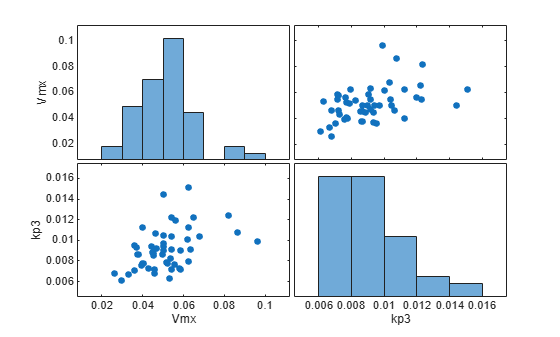Simulate the scenarios using the same SimFunction you created previously. You do not need to create a new SimFunction object even though the Scenarios object has been updated.

sd2 = f(sObj,24);
sbioplot(sd2);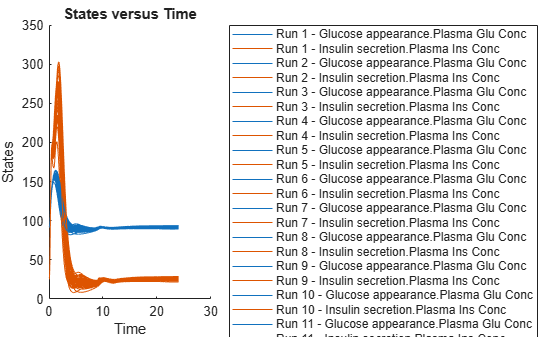By default, SimBiology uses the random sampling method. You can change it to the Latin hypercube sampling (or sobol or halton) for a more systematic space-filling approach.

entry2struct = getEntry(sObj,2)
entry2struct = struct with fields:
Name: {'Vmx'  'kp3'}
Content: [2×1 prob.LognormalDistribution]
Number: 100
RankCorrelation: [2×2 double]
Covariance: []
SamplingMethod: 'random'

entry2struct.SamplingMethod = 'lhs'
entry2struct = struct with fields:
Name: {'Vmx'  'kp3'}
Content: [2×1 prob.LognormalDistribution]
Number: 100
RankCorrelation: [2×2 double]
Covariance: []
SamplingMethod: 'lhs'

You can now use the updated structure to modify entry 2.

updateEntry(sObj,2,entry2struct)
ans =
Scenarios (100 scenarios)

Name           Content            Number
____    ______________________    ______

Entry 1         dose    SimBiology dose            1
x (Entry 2.1    Vmx     Lognormal distribution     100
+ Entry 2.2)    kp3     Lognormal distribution     100

Visualize the sample values.

sTbl2 = generate(sObj);
[s,ax,bigax,h,hax] = plotmatrix([sTbl2.Vmx,sTbl2.kp3]);
ax(1,1).YLabel.String = "Vmx";
ax(2,1).YLabel.String = "kp3";
ax(2,1).XLabel.String = "Vmx";
ax(2,2).XLabel.String = "kp3";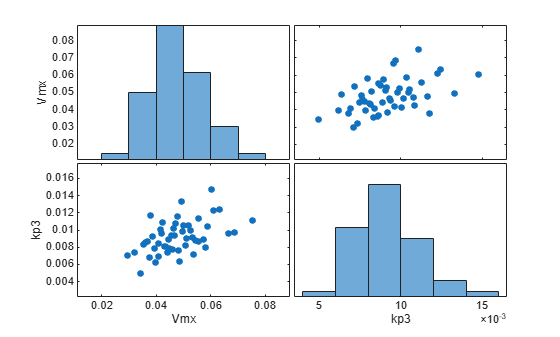Simulate the scenarios.

sd3 = f(sObj,24);
sbioplot(sd3);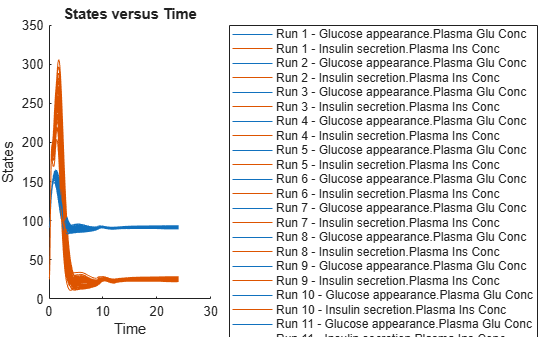Restore warning settings.

warning(warnSettings);